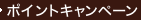•### ＩＴＳにおけるウェーブレット解析Wavelets in Intelligent Transportation Systems

• 在庫がございません。海外の書籍取次会社を通じて出版社等からお取り寄せいたします。
通常6～9週間ほどで発送の見込みですが、商品によってはさらに時間がかかることもございます。
重要ご説明事項
1. 納期遅延や、ご入手不能となる場合がございます。
2. 複数冊ご注文の場合、分割発送となる場合がございます。
3. 美品のご指定は承りかねます。
• ≪洋書のご注文につきまして≫ 「海外取次在庫あり」および「国内仕入れ先からお取り寄せいたします」表示の商品でも、納期の目安期間内にお届けできないことがございます。あらかじめご了承ください。

• 製本 Hardcover:ハードカバー版／ページ数 224 p.
• 言語 ENG
• 商品コード 9780470867426
• DDC分類 388.312

### 基本説明

Focuses on the models and algorithms for traffic incident detection.

### Full Description

This book shows how wavelets can be used to enhance computational intelligence for chaotic and complex pattern recognition problems. By integrating wavelets with other soft computing techniques such as neurocomputing and fuzzy logic, complicated and noisy pattern recognition problems can be solved effectively. The book focuses on applications in intelligent transportation systems (ITS) where a number of very complicated pattern recognition problems have eluded researchers over the past few decades. Advancing the frontiers of computational intelligence, this book describes ingenious computational models based on novel problem solving and computing techniques such as Case-Based Reasoning, Neurocomputing, and Wavelets, and presents examples to illustrate their importance and use.It presents a multi-paradigm intelligent systems approach to the freeway traffic incident detection and construction work zone management problems. It advocates application and integration of wavelets, neural networks and fuzzy logic for modeling the complex traffic flow behaviors leading to effective and efficient control and management solutions.It presents efficient, reliable, and robust algorithms for automatic detection of incidents on freeways. "Wavelets in Intelligent Transportation Systems" is an invaluable resource for computational intelligence researchers and transportation engineers involved in the application of advanced computational techniques for ITS.

### Contents

Preface. Acknowledgment. About the Authors. 1. Introduction. 2. Introduction to Wavelet Analysis. 2.1 Introduction. 2.2 Basic Concept of Wavelets and Wavelet Analysis. 2.2.1 What is a Wavelet?. 2.2.2 Wavelet Analysis. 2.2.3 Types of Wavelets and Wavelet Transforms. 2.3 Mathematical Foundations. 2.3.1 Sets and Spaces. 2.3.2 Sequence and Function Spaces. 2.3.3 Independent and Basis Sets. 2.3.4 Metric, Normed and Inner Product Spaces. 2.3.5 The L2(R) and L2(Z) Spaces. 2.3.6 Orthogonality. 2.4 The Discrete Wavelet Transform (DWT). 2.5 Multi-resolution Analysis. 2.6 Wavelet Bases. 2.6.1 Constructing Wavelet Bases. 2.6.2 Example Wavelet Systems. 2.7 Computing the DWT. 2.7.1 Pyramid Algorithm. 2.7.2 Practical Considerations. 3. Feature Extraction for Traffic Incident Detection Using Wavelet Transform and Linear Discriminant Analysis. 3.1 Introduction. 3.2 Incident Detection Algorithms. 3.3 Discrete Wavelet Transform (DWT) of Traffic Signals. 3.4 Linear Discriminant Analysis (LDA). 3.5 Data Acquisition. 3.6 Results. 4. Adaptive Conjugate Neural Network-Wavelet Model for Traffic Incident Detection. 4.1 Introduction. 4.2 Improving Traffic Incident Detection. 4.3 Adaptive Conjugate Gradient Neural Network Model. 4.4 Incident Detection Results Using Various Approaches. 4.4.1 LDA. 4.4.2 DWT and LDA. 4.4.3 ACGNN. 4.4.4 DWT, LDA, and ACGNN. 4.5 Effect of Data Filtering Using DWT. 4.6 Relative Contribution of DWT and LDA for Feature Extraction. 4.7 Effects of Freeway Geometry on Incident Detection. 4.7.1 Effect of Curvature. 4.7.2 Effect of Number of Lanes. 4.8 Conclusion. 5. Enhancing Fuzzy Neural Network Algorithms Using Neural Networks. 5.1 Introduction. 5.2 Discrete Wavelet Transform. 5.3 Architecture. 5.4 Training of the Network. 5.5 Filtering of the Traffic Data Using DWT. 5.6 Incident Detection Results. 6. Fuzzy-Wavelet Radial Basis Function Neural Network Model for Freeway Incident Detection. 6.1 Introduction. 6.2 A New Traffic Incident Detection Methodology. 6.3 Selection of Type and Number of Traffic Data. 6.4 Wavelet-Based De-noising. 6.5 Fuzzy Data Clustering. 6.6 Radial Basis Function Neural Network Classifier. 6.7 Fuzzy-Wavelet RBFNN Model for Incident Detection. 6.8 Example. 6.8.1 Training of Algorithm. 6.8.2 Testing of Algorithm Using Simulated Data. 6.8.3 Testing of Algorithm Using Real Data. 6.9 Conclusion. 7. Comparison of Fuzzy-Wavelet RBFNN Freeway Incident Detection Model with California Algorithm. 7.1 Introduction. 7.2 California Algorithm #8. 7.3 Evaluation of the Model. 7.3.1 Introduction. 7.3.2 Evaluation Criteria. 7.3.3 Traffic Data. 7.3.4 Training and Calibration. 7.3.5 First Simulation Test - Parametric Evaluation. 7.3.6 Second Simulation Test - Freeway with On- and Off-Ramps. 7.3.7 Test Using Real Data. 7.4 Concluding Remarks. 8. Incident Detection Algorithm Using Wavelet Energy Representation of Traffic Patterns. 8.1 Introduction. 8.2 Freeway Incident Detection and Patterns in Traffic Flow. 8.2.1 Single-Station Versus Two-Station Incident Detection Approaches. 8.2.2 Upstream and Downstream Flow Patterns. 8.3 Discrete Wavelet Transform and Signal Energy. 8.4 Traffic Pattern Feature Enhancement and De-noising. 8.5 Pattern Classification Using Radial Basis Function Neural Networks. 8.6 Wavelet Energy Freeway Incident Detection Algorithm. 8.7 Model Testing. 8.7.1 Introduction. 8.7.2 Training. 8.7.3 First Test Using Simulated Data: Two-lane Freeway. 8.7.4 Second Test Using Simulated Data: Three-lane Freeway. 8.7.5 Third Test Using Simulated Data: Compression Waves. 8.7.6 Fourth Test Using Real Data: FSP Project's I-880 Database. 8.7.7 Result Summary and Comparison. 8.8 Conclusion. 9. Parametric Evaluation of the Wavelet Energy Freeway Incident Detection Algorithm. 9.1 Introduction. 9.2 Factors to Consider in Rural Freeway Incident Detection. 9.3 Evaluation and Parametric Investigation. 9.3.1 Goals. 9.3.2 Data. 9.3.3 Training and Calibration. 9.4 Parametric Evaluation Using Simulated Data on Typical Urban Freeways. 9.5 False Alarm Performance in the Vicinity of On- and Off-Ramps. 9.6 Evaluation on Rural Freeways. 9.7 Evaluation Using Real Data. 9.8 Performance Summary and Conclusion. 10. Case-Based Reasoning Model for Work Zone Traffic Management. 10.1 Introduction. 10.2 Work Zones and Traffic Management. 10.3 Case-Based Reasoning. 10.4 Objectives. 10.5 Scope and Categorization of Parameters. 10.5.1 Work Zone Type. 10.5.2 Work Zone Layout. 10.5.3 Work Characteristics. 10.5.4 Traffic flow characteristics. 10.5.5 Phases of Work. 10.5.6 Traffic Control Measures. 10.5.7 Road User Cost. 10.6 A Four-Set Case Model for the Work Zone Traffic Management Domain. 10.7 Hierarchical Object-Oriented Case Model. 10.8 Case Representation. 10.9 Similarity Measures. 10.10 Case Retrieval. 10.11 Creation of the Case Base. 10.12 Creation of Work Zone Traffic Control Plans Using the CBR System. 10.13 Illustrative Examples. 10.13.1 Example 1. 10.13.2 Example 2. 10.13.3 Example 3. 10.14 Conclusion. 11. Mesoscopic-Wavelet Freeway Work Zone Flow and Congestion Model. 11.1 Introduction. 11.2 Macroscopic Models. 11.3 Microscopic Models. 11.4 A Mesoscopic Flow Model for a Freeway Work Zone. 11.5 Traffic Feature Enhancement Using Discrete Wavelet Transform. 11.6 Concluding Remarks. References. Index.